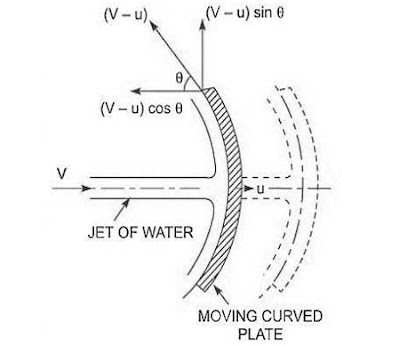# The curved plate moving of jet - Ishwaranand

## Force on the curved plate when the plate is moving in the direction of the jet

Let a jet of water strikes a curved plate at the centre of the plate which is moving with a uniform velocity in the direction of the jet as shown in Fig.Image Source ~ Crafted With ©Ishwaranand - 2020 ~ Image by ©Ishwaranand
Fig. Jet striking a curved moving plate
Let
v = Absolute velocity of jet,
a = Area of a jet,
u = Velocity of the plate in the direction of the jet.

The relative velocity of the jet of water or the velocity with which jet strikes the curved plate
= (v - u)
If the plate is smooth and the loss of energy due to impact of a jet is zero, then the velocity with which the jet will be leaving the curved vane
= (v - u)
This velocity can be resolved into two components, one in the direction of the jet and other perpendiculars to the direction of the jet.

Component of the velocity in the direction of the jet
= - (v - u) cosθ
(-ve sign is taken as at the outlet, the component is in the opposite direction of the jet).

Component of the velocity in the direction perpendicular to the direction of the jet
= (v - u) sinθ
Mass of the water striking the plate
= ρa x velocity with which jet strikes the plate
= ρa (v –u)

Force exerted by a jet of water on the curved plate in the direction of the jet, Fx

Fx = Mass striking per second x [Initial velocity with which jet strikes the plate in the direction of jet – Final velocity]
= ρa(v – u) [(v – u) – (– (v – u) cosθ)]
= ρa(v – u) [(v – u) + (v – u) cosθ]
= ρa(v – u)^2 [ 1 + cosθ]

Work is done by the jet on the plate per second

= Fx x Distance travelled per second in the direction of x
= Fx x u
= ρa(v – u)^2 [ 1 + cosθ] x u
= ρa(v – u)^2 u [ 1 + cosθ]
Ex. A jet of water of diameter 7.5 cm strikes a curved plate at its centre with a velocity of 20 m/s. This curved plate is moving by a velocity of 8 m/s in the direction from the jet. Each jet is deflected through an angle of 165°. Considering the plate smooth,
Find
1. Force exerted at the plate into the direction from the jet,
2. Power of the jet, and
3. The efficiency of the jet.
Given
The diameter of the jet,
d = 7.5 cm
= 0.075 m
Area,
a = (π/4) (0.075)^2
= 0.004417
The velocity of the jet,
v = 20 m/s
The velocity of the plate,
u = 8 m/s
The angle of deflection of the jet
= 165°

Solution
Angle made by the relative velocity at the outlet of the plate,
θ = 180° - 165°
= 15°
Force exerted by the jet on the plate in the direction of the jet is given by,
Fx = ρa (v - u)^2 (1 + cosθ)
= 1000 x 0.004417 x (20 - 8)^2 [1 + cos15°]
= 1250.38 N
Work done to the jet on this plate is /sec
= Fx x u
= 1250.38 x 8
= 10003.04 N m/s
Power of the jet
= 10003.04/1000
= 10 kW
= Output / Input
= (Work done by jet/sec) / ( Kinetic energy of jet/sec )
= (1250.38 x 8) / 1/2(pav^3)
= (1250.38 X 8) / 1/2(1000 x 0.004417 x 20^3)
= 56.4%